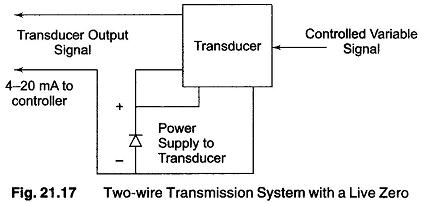## Choice of Electronic Signal Transmission:

The range of air pressure used for Signal Transmission in pneumatic system is limited by the dynamic operating range of the pneumatic devices used. But the choice of electrical transmission is much wider. Since either ac or dc current may be selected. In addition, a choice may be made between a voltage or a current level, the range of signal levels has also to be decided.

A dc current transmission has the advantage over ac transmission in that only the resistance of the transmission lines need to be considered. The effects of line inductance and capacitance can be ignored. Certain types of measuring device operate on ac current, but the output from these can be converted into dc by relatively simple means.

A current rather than a voltage is normally used as the method of signal transmission, since the effects of line resistance have very little effect on the accuracy of the information transmitted. An output current is obtained from transducers and controllers by providing current feedback in the amplifier used. Wherever necessary, the current signal can be converted into a voltage signal by connecting a resistor in the line.

The range of current values used varies widely, typically ranges being 0 — 10 mA, 0 — 20 mA, and 4 — 20 mA. The live zero of 4 mA permits transducers to use the line characteristic as a source of power for its amplifier as shown in Fig. 21.17.By this means, a  transmission system is obtained. A disadvantage of the line zero is that the controlled variable zero does not correspond to a true zero of the transmitted current. This presents problems where mathematical operations, e.g. a square root function, have to be carried out on the current.

Scroll to Top